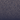# The Last Plateau

For life is always uphill. You must keep ascending, even when you feel you've reached The Last Plateau.## In a nutshell

• Generate a uniformly distributed set of n points `(x, y)`
• Get the Voronoi diagram from the set of points.
• For each cell in the diagram:

• Assign a height value `z = f(x, y)`
• Transform to simulate a 3D Visualization. Now each cell is a cell prism.
• Assign a color `c = f(θ)`to each cell prism face, where

• `θ` is the angle of the prism face with respect to the X axis